## Strong Acid-Strong Base Titrations

A strong acid will react with a strong base to form a neutral (pH = 7) solution.

### Learning Objectives

Calculate the concentration of an unknown strong acid given the amount of base necessary to titrate it.

### Key Takeaways

#### Key Points

• An acid – base titration is used to determine the unknown concentration of an acid or base by neutralizing it with an acid or base of known concentration.
• Neutralization is the reaction between an acid and a base, producing a salt and a neutralized base.
• A strong acid yields a weak conjugate base (A), so a strong acid is also described as an acid whose conjugate base is a much weaker base than water.

#### Key Terms

• strong acid: A strong acid is one that completely ionizes (dissociates) in water; in other words, one mole of a strong acid (HA) dissolves in water yielding one mole of H+ and one mole of the conjugate base, A−.
• titration: The determination of the concentration of some substance in a solution by slowly adding measured amounts of some other substance (normally using a burette) until a reaction is shown to be complete—for instance, by the color change of an indicator.
• stoichiometry: The calculation of relative quantities or reactants and products in chemical reactions.
• strong base: A strong base is a basic chemical compound that is able to deprotonate very weak acids in an acid-base reaction. Common examples of strong bases are the hydroxides of alkali metals and alkaline earth metals, such as NaOH and Ca(OH)2. Very strong bases are even able to deprotonate very weakly acidic C–H groups in the absence of water.

An acid-base titration is used to determine the unknown concentration of an acid or base by neutralizing it with an acid or base of known concentration. Using the stoichiometry of the reaction, the unknown concentration can be determined. It makes use of the neutralization reaction that occurs between acids and bases and the knowledge of how acids and bases will react if their formulas are known.

### Stages of a Strong Acid-Strong Base Titration

A strong acid- strong base titration is performed using a phenolphthalein indicator. Phenolphtalein is chosen because it changes color in a pH range between 8.3 – 10. It will appear pink in basic solutions and clear in acidic solutions. In the case of a strong acid-strong base titration, this pH transition would take place within a fraction of a drop of actual neutralization, since the strength of the base is high.

The addition of reactants is done from a burette. The reactant of unknown concentration is deposited into an Erlenmeyer flask and is called the analyte. The other reactant of known concentration remains in a burette to be delivered during the reaction. It is known as the titrant. The indicator—phenolphthalein, in this case—has been added to the analyte in the Erlenmeyer flask.Titration: Titration of an acid-base system using phenolphthalein as an indicator.

Neutralization is the reaction between an acid and a base, producing a salt and neutralized base. For example, hydrochloric acid and sodium hydroxide form sodium chloride and water:

$\text{HCl} (\text{aq}) + \text{NaOH} (\text{aq}) \rightarrow \text{H}_2\text{O} (\text{l}) + \text{NaCl} (\text{aq})$

Neutralization is the basis of titration. A pH indicator shows the equivalence point —the point at which the equivalent number of moles of a base have been added to an acid. It is often wrongly assumed that neutralization should result in a solution with pH 7.0; this is only the case in a strong acid and strong base titration.

### Example:

What is the unknown concentration of a 25.00 mL HCl sample that requires 40.00 mL of 0.450 M NaOH to reach the equivalence point in a titration?

$\text{HCl} (\text{aq}) + \text{NaOH} (\text{aq}) \rightarrow \text{H}_2\text{O} (\text{l}) + \text{NaCl} (\text{aq})$

Step 1: First calculate the number of moles of NaOH added during the titration.

$0.450 \frac{\text{moles}}{\text{L}} \text{NaOH} \times0.0400 \text{L} = 0.018\ \text{moles}\ \text{NaOH}$

Step 2: Use stoichiometry to figure out the moles of HCl in the analyte.

The mole ratio between HCl and NaOH in the balanced equation is 1:1.

$0.018 \ \text{moles} \ \text{NaOH} \times \frac{1\ \text{mole} \ \text{HCl}}{1\ \text{mole}\ \text{NaOH}} = 0.018 \ \text{moles} \ \text{HCl}$

Step 3: Calculate the molar concentration of HCL in the 25.00 mL sample.

Molarity of HCl = $\frac {0.018 \ \text{moles} \ \text{HCl}}{0.025 \ \text{L} \ \text{HCl}} = 0.72 \ \text{Molar} \ \text{HCl}$

## Weak Acid-Strong Base Titrations

A weak acid will react with a strong base to form a basic (pH > 7) solution.

### Learning Objectives

Distinguish a weak acid-strong base titration from other types of titrations.

### Key Takeaways

#### Key Points

• In an acid – base titration, the titration curve reflects the strengths of the corresponding acid and base.
• If one reagent is a weak acid or base and the other is a strong acid or base, the titration curve is irregular, and the pH shifts less with small additions of titrant near the equivalence point.
• Acid-base titrations depend on the neutralization between an acid and a base when mixed in solution.
• The endpoint and the equivalence point are not exactly the same: the equivalence point is determined by the stoichiometry of the reaction, while the endpoint is just the color change from the indicator.

#### Key Terms

• stoichiometry: The study and calculation of quantitative (measurable) relationships of the reactants and products in chemical reactions (chemical equations).
• buffer: A solution used to stabilize the pH (acidity) of a liquid.
• pH: The negative of the logarithm to base 10 of the concentration of hydrogen ions, measured in moles per liter; a measure of acidity or alkalinity of a substance, which takes numerical values from 0 (maximum acidity) through 7 (neutral) to 14 (maximum alkalinity).
• equivalence point: The point in a chemical reaction at which chemically equivalent quantities of acid and base have been mixed.

Titrations are reactions between specifically selected reactants—in this case, a strong base and a weak acid. A titration curve reflects the strength of the corresponding acid and base, showing the pH change during titration. The titration curve demonstrating the pH change during the titration of the strong base with a weak acid shows that at the beginning, the pH changes very slowly and gradually. This indicates the formation of a buffer system as the titration approaches the equivalence point.

At the equivalence point and beyond, the curve is typical of a titration of, for example, NaOH and HCl. When the NaOH is in excess, the pH change is the same as in any system dominated by NaOH.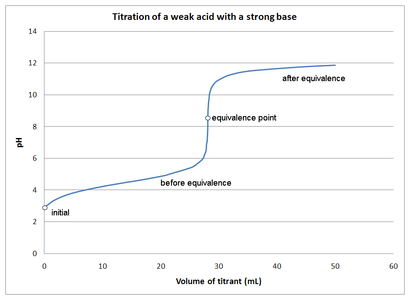Titration of a weak Acid with a strong base: This figure depicts the pH changes during a titration of a weak acid with a strong base.

The initial pH of the solution at the beginning of the titration is approximately that of the weak acid in water. At the equivalence point, all of the weak acid is neutralized and converted to its conjugate base (the number of moles of H+ = added number of moles of OH). However, the pH at the equivalence point does not equal 7. This is due to the production of conjugate base during the titration. The resulting solution is slightly basic. The endpoint and the equivalence point are not exactly the same: the equivalence point is determined by the stoichiometry of the reaction, while the endpoint is just the color change from the indicator.

### Example:

The titration of acetic acid (HC2H3O2) with NaOH.

$\text{HC}_2\text{H}_3\text{O}_2 + \text{OH}^- \rightarrow \text{H}_2\text{O} + \text{C}_2\text{H}_3\text{O}_2^-$

During this titration, as the OH reacts with the H+ from acetic acid, the acetate ion (C2H3O2) is formed. This conjugate base reacts with water to form a slightly basic solution.

$\text{C}_2\text{H}_3\text{O}_2^- + \text{H}_2\text{O} \rightarrow \text{HC}_2\text{H}_3\text{O}_2 + \text{OH}^-$

## Strong Acid-Weak Base Titrations

A strong acid will react with a weak base to form an acidic (pH < 7) solution.

### Learning Objectives

Recall that strong acid-weak base titrations can be performed with either serving as the titrant.

### Key Takeaways

#### Key Points

• The titration is typically performed as an acid into base.
• Following the titration with a pH meter in real time generates a curve showing the equivalence point.
• If the approximate pH of the equivalence point is known, a colorimetric indicator can be used in the titration.

#### Key Terms

• buffer: A solution used to stabilize the pH (acidity) of a liquid.
• titration: The determination of the concentration of some substance in a solution by slowly adding measured amounts of some other substance (normally using a burette) until a reaction is shown to be complete—for instance, by the color change of an indicator.
• equivalence point: The point in a chemical reaction at which chemically equivalent quantities of acid and base have been mixed.

An example of a strong acid – weak base titration is the reaction between ammonia (a weak base) and hydrochloric acid (a strong acid) in the aqueous phase:

$\text{NH}_3 (\text{aq}) + \text{HCl} (\text{aq}) \rightarrow {\text{NH}_4^+}(\text{aq}) + \text{Cl}^-(\text{aq})$

The acid is typically titrated into the base. A small amount of the acid solution of known concentration is placed in the burette (this solution is called the titrant ). A known volume of base with unknown concentration is placed into an Erlenmeyer flask (the analyte), and, if pH measurements can be obtained via electrode, a graph of pH vs. volume of titrant can be made (titration curve).

In the case of titrating the acid into the base for a strong acid-weak base titration, the pH of the base will ordinarily start high and drop rapidly with the additions of acid. As the equivalence point is approached, the pH will change more gradually, until finally one drop will cause a rapid pH transition through the equivalence point. If a chemical indicator is used—methyl orange would be a good choice in this case—it changes from its basic to its acidic color.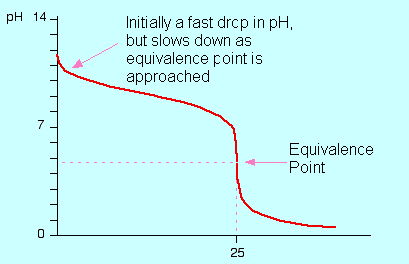Titration of a weak base with a strong acid: A depiction of the pH change during a titration of HCl solution into an ammonia solution. The curve depicts the change in pH (on the y-axis) vs. the volume of HCl added in mL (on the x-axis).

In strong acid-weak base titrations, the pH at the equivalence point is not 7 but below it. This is due to the production of a conjugate acid during the titration; it will react with water to produce hydronium (H3O+) ions.

In the example of the titration of HCl into ammonia solution, the conjugate acid formed (NH4+) reacts as follows:

$\text{NH}_4^+ + \text{H}_2\text{O} \rightarrow \text{H}_3\text{O}^+ + \text{NH}_3$

## Polyprotic Acid Titrations

Polyprotic acids, also known as polybasic acids, are able to donate more than one proton per acid molecule.

### Learning Objectives

Recall the general shape of a pH vs equivalents graph generated by titrating a polyprotic acid.

### Key Takeaways

#### Key Points

• A diprotic acid contains two protons (H+) and can produce two hydrogen ions in solution.
• Certain types of polyprotic acids have more specific names, such as diprotic acid (two potential protons to donate) and triprotic acid (three potential protons to donate).
• Although the subsequent loss of each sequential hydrogen ion is increasingly less favorable, all of the conjugate bases are present in solution.

#### Key Terms

• titration: The determination of the concentration of some substance in a solution by slowly adding measured amounts of some other substance (normally using a burette) until a reaction is shown to be complete—for instance, by the color change of an indicator.
• monoprotic acid: One that is able to donate one hydrogen ion per molecule during the process of dissociation.
• polybasic: Containing two or more replaceable hydrogen atoms.
• polyprotic: Of an acid (or a base) that can donate (or accept) more than one proton; polybasic.

### Monoprotic Acids

Monoprotic acids are acids able to donate one proton per molecule during the process of dissociation (sometimes called ionization) as shown below (symbolized by HA):

${ \text{HA} }_{ (\text{aq}) }\quad +\quad { \text{H} }_{ 2 }{ \text{O} }_{ (\text{l}) }\quad \rightleftharpoons \quad { \text{H} }_{ 3 }{ \text{O} }_{ (\text{aq}) }^{ + }\quad +\quad { \text{A} }_{ (\text{aq}) }$

Common examples of monoprotic acids in mineral acids include hydrochloric acid (HCl) and nitric acid (HNO3). On the other hand, for organic acids the term mainly indicates the presence of one carboxylic acid group, and sometimes these acids are known as monocarboxylic acid.

### Polyprotic Acids

Polyprotic acid are able to donate more than one proton per acid molecule, in contrast to monoprotic acids that only donate one proton per molecule. Certain types of polyprotic acids have more specific names, such as diprotic acid (two potential protons to donate) and triprotic acid (three potential protons to donate).

For example, oxalic acid, also called ethanedioic acid, is diprotic, having two protons to donate.Oxaliic Acid Showing consecutive losses of H+: This image shows how Oxalic Acid will lose two protons in successive dissociations.

If a dilute solution of oxalic acid were titrated with a sodium hydroxide solution, the protons would react in a stepwise neutralization reaction.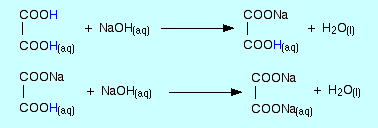Neutralization of a diprotic acid: Oxalic acid undergoes stepwise neutralization by sodium hydroxide solution.

If the pH of this titration were recorded and plotted against the volume of NaOH added, a very clear picture of the stepwise neutralization emerges, with very distinct equivalence points on the titration curves.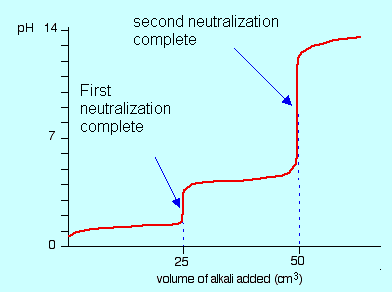Titration curve for diprotic acid: The titration of dilute oxalic acid with sodium hydroxide (NaOH) shows two distinct neutralization points due to the two protons.

Oxalic acid is an example of an acid able to enter into a reaction with two available protons, having different Ka values for the dissociation (ionization) of each proton.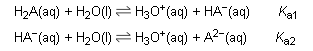A diprotic acid dissociation: The diprotic acid has two associated values of Ka, one for each proton.

Likewise, a triprotic system can be envisioned. Each reaction proceeds with its unique value of Ka.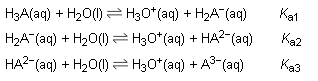Triprotic acid dissociation: Triprotic acids can make three distinct proton donations, each with a unique Ka.

An example of a triprotic acid is orthophosphoric acid (H3PO4), usually just called phosphoric acid. All three protons can be successively lost to yield H2PO4, then HPO42-, and finally PO43- the phosphate ion. Another example of a triprotic acid is citric acid, which can successively lose three protons to finally form the citrate ion.

## Acid-Base Indicators

An indicator is a weak acid (or a weak base) that has different colors in its dissociated and undissociated states.

### Learning Objectives

Explain which, of a given series, would be the best acid-base indicator for a given titration.

### Key Takeaways

#### Key Points

• In general, a molecule that changes color with the pH of the environment it is in can be used as an indicator.
• In the reaction $\text{HIn}\rightleftharpoons { \text{H} }^{ + } +{ \text{In} }^{ - }$, adding acid shifts the indicator equilibrium to the left.
• In the reaction $\text{HIn}\rightleftharpoons { \text{H} }^{ + } +{ \text{In} }^{ - }$, adding base shifts the indicator equilibrium to the right.
• For optimal accuracy, the color difference between the two colored species should be as clear as possible, and the narrower the pH range of the color change the better.

#### Key Terms

• pH indicator: An acid-base indicator.
• titration: The determination of the concentration of some substance in a solution by slowly adding measured amounts of some other substance (normally using a burette) until a reaction is shown to be complete—for instance, by the color change of an indicator
• indicator: A halochromic chemical compound that is added in small amounts to a solution so that the pH (acidity or basicity) of the solution can be determined visually.
• pH: The negative of the logarithm to base 10 of the concentration of hydrogen ions, measured in moles per liter; a measure of acidity or alkalinity of a substance, which takes numerical values from 0 (maximum acidity) through 7 (neutral) to 14 (maximum alkalinity).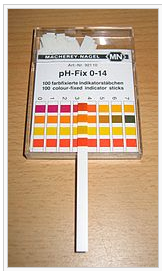pH measuring strips: pH can be determined to a reasonable level of accuracy by treating a strip with the solution to be tested and then observing the color sequence on the treated area.

There are many methods to determine the pH of a solution and to determine the point of equivalence when mixing acids and bases. These methods range from the use of litmus paper, indicator paper, specifically designed electrodes, and the use of colored molecules in solution. Other than the electrodes, all of the methods are visual and rely on some fundamental changes that occur in a molecule when the pH of its environment changes. In general, a molecule that changes color with the pH of the environment it is in can be used as an indicator.

In the equation:

$\text{HIn} \rightleftharpoons { \text{H} }^{ + }+ { \text{In} }^{ - }$

the weak acid HIn is shown in equilibrium with its ionized anion In. In this reaction, adding acid shifts the indicator equilibrium to the left. Conversely, adding a base shifts the indicator equilibrium to the right.

In the case of the indicator methyl orange, the HIn is colored red and the ionized In form is yellow.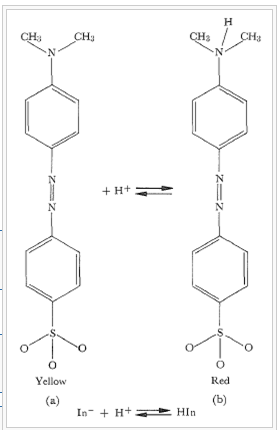Methyl orange: The molecule methyl orange is commonly used as an indicator in acid-base equilibrium reactions. In base form, on the left in the figure, the color is yellow. Adding a proton yields the structure on the right, colored red. Note that this color change occurs over the pH range from approximately 3-4.

In this example:

${ \text{K} }_{ \text{a} }\quad =\quad \frac { \left[ { \text{H} }^{ + } \right] \left[ { \text{In} }^{ - } \right] }{ \left[ \text{HIn} \right] }$

For methyl orange, Ka = 1.6 X 10-4 and pKa = 3.8. The neutral (red) and dissociated (yellow) forms of the indicator are present at equal concentrations when the pH = 3.8. The eye is sensitive to color changes over a range of concentration ratios of approximately 100 or over two pH units. Below pH 2.8, a solution containing methyl orange is red; above approximately 4.8, it is clearly yellow.

pH indicators are frequently employed in titrations in analytical chemistry and biology to determine the extent of a chemical reaction. Because of the subjective choice (determination) of color, pH indicators are susceptible to imprecise readings. For applications requiring precise measurement of pH, a pH meter is frequently used. Sometimes a blend of different indicators is used to achieve several smooth color changes over a wide range of pH values. These commercial indicators (e.g., universal indicator and Hydrion papers) are used when only rough knowledge of pH is necessary. Indicators usually exhibit intermediate colors at pH values inside a specific transition range. For example, phenol red exhibits an orange color between pH 6.8 and pH 8.4. The transition range may shift slightly depending on the concentration of the indicator in the solution and on the temperature at which it is used.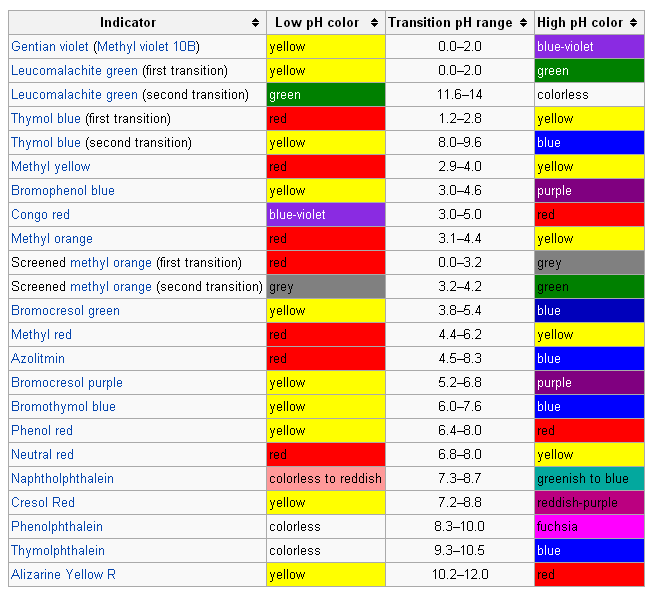Common acid-base indicators: Common indicators for pH indication or titration endpoints is given, with high, low, and transition pH colors.

When viewed on the pH scale itself, the color transitions as determined by their transition ranges becomes clearer and the context of the indicator sensitivity over ranges of pH is laid out more informatively.

### Example:

In the titration of a weak acid with a strong base, which indicator would be the best choice?

A. Methyl Orange

B. Bromocresol Green

C. Phenolphtalein

The correct answer is C. In the titration of a weak acid with a strong base, the conjugate base of the weak acid will make the pH at the equivalence point greater than 7. Therefore, you would want an indicator to change in that pH range. Both methyl orange and bromocresol green change color in an acidic pH range, while phenolphtalein changes in a basic pH.